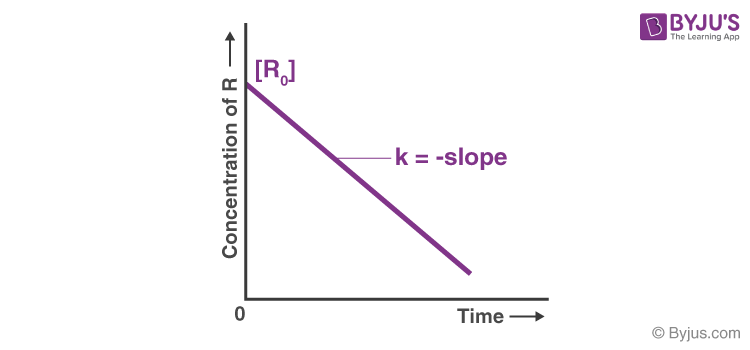Checkout JEE MAINS 2022 Question Paper Analysis : Checkout JEE MAINS 2022 Question Paper Analysis :

# Chemistry Practical Class 12 Reaction of Iodide ion with Hydrogen peroxide at room temperature using different concentrations of Iodide ions Viva Questions with Answers

Q1. What is the concentration of KI solution used in this experiment?

Q2. What is the concentration of Na2S2O3 solution used in this experiment?

Q3. What is the percentage of hydrogen peroxide used in this experiment?

Q4. For a reaction of iodide ion with hydrogen peroxide at room temperature, what will be the order of reaction concerning Iodide ion?

Q5. What is the colour of the starch iodine complex formed?

Q6. What is the effect of concentration on the rate of a reaction?

Answer: The rate of reaction increases with concentration. A higher concentration of a reactant leads to more collisions of reactant in a specific time, thereby increasing the reaction rate.

Q7. Why are few reactions very fast?

Answer: Because it has very less activation energy.

Q8. What is the unit of first-order reaction?

Answer: Mol -1 L -1 sec -1

Q9. What is the effect of a catalyst on the activation energy and rate of reaction?

Answer: A catalyst provides an alternate pathway by reducing the activation energy of the reaction, thereby increasing the rate of reaction.

Q10. Can the order of reaction be fractional

Answer: Yes, the reaction order can be fractional.

For example, CH3CHO → CH4 + CO.

The order of the above reaction is 3/2.

Q11. What are the factors that affect the rate of a reaction?

Answer: Various factors can affect the rate of a chemical reaction.

• Temperature
• Concentration of the reactant
• Physical state
• Catalyst

Q12. What is a complex reaction?

Answer: A reaction involving more than one step is known as a complex reaction.

Q13. What is the temperature coefficient of a reaction?

Answer: The temperature coefficient of a reaction is the ratio of rate constants at two temperatures differing by 10°. Its value is generally equal to 2.

Q14. What is the unit of rate constant for the first-order reaction?

Q15. What is chemical kinetics?

Answer: Chemical kinetics is the branch of physical chemistry that deals with the rate of a chemical reaction and its mechanism.

Q16. What is the rate of reaction?

Answer: The reaction rate is defined as a change in molar concentration of reactant or product per unit time.

Q17. What is threshold energy?

Answer: Threshold energy is the minimum kinetic energy that all colliding molecules must have to make effective collisions between two reactant molecules.

Q18. “For an exothermic reaction, the activation energy for the forward reaction should be less than that for the backward reaction.” Is this statement true or false?

Answer: Yes, the above statement is true.

Q19. On increasing the concentration of reactants, the reaction rate does not alter. What can you say about the order of the reaction?

Answer: It is a zero-order reaction.

Q20. How will you plot a graph between the concentration and time of zero-order reaction?

Answer:Download Class 12 Chemistry Viva questions on the Reaction of Iodide ions with Hydrogen peroxide at room temperature using different concentrations of Iodide ions by clicking on the button below.